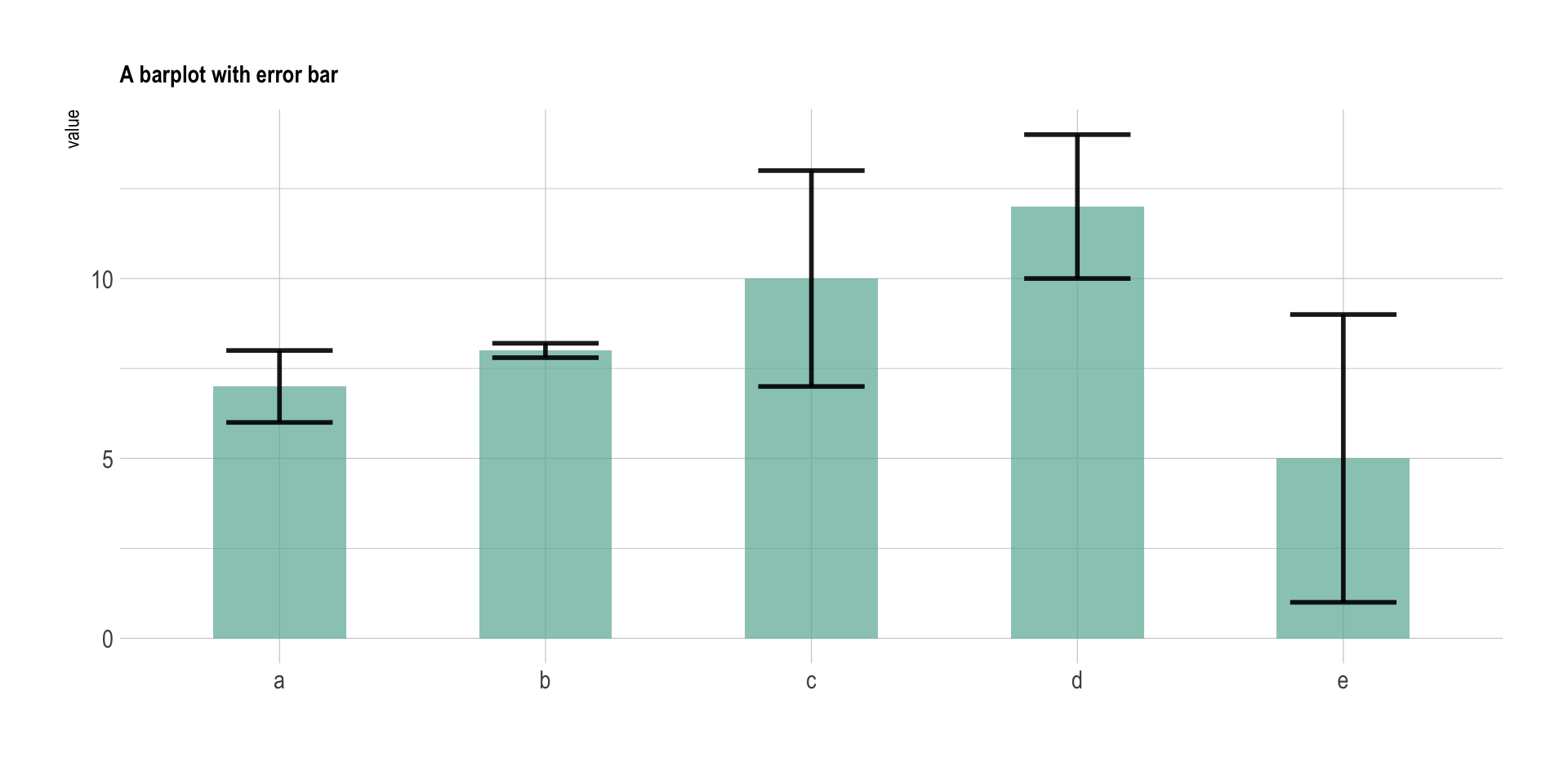Error bars give a general idea of how precise a measurement is, or conversely, how far from the reported value the true (error free) value might be. If the value displayed on your barplot is the result of an aggregation (like the mean value of several data points), you may want to display error bars.

``````# Libraries
library(tidyverse)
library(hrbrthemes)
library(viridis)
library(patchwork)

# create dummy data
data <- data.frame(
name=letters[1:5],
value=sample(seq(4,15),5),
sd=c(1,0.2,3,2,4)
)

# Plot
ggplot(data) +
geom_bar( aes(x=name, y=value), stat="identity", fill="#69b3a2", alpha=0.7, width=0.5) +
geom_errorbar( aes(x=name, ymin=value-sd, ymax=value+sd), width=0.4, colour="black", alpha=0.9, size=1) +
theme_ipsum() +
theme(
legend.position="none",
plot.title = element_text(size=11)
) +
ggtitle("A barplot with error bar") +
xlab("")``````In the graphic above 5 groups are reported. The bar heights represent their mean value. The black error bar gives information on how the individual observations are dispersed around the average. For instance, it appears that measurements in group `B` are more precise than in group `E`.

# Error bars hide information

The first issue with error bars is that they hide information. Here is a figure from a paper in PLOS Biology. It illustrates that the full data may suggest different conclusions than the summary statistics. The same barplot with error bars (left) can represent several situations. Both groups can have the same kind of distribution (`B`), one group can have outliers (`C`), one group can have a bimodal distribution (`D`), or groups can have unequal sample sizes: